DAY 26
0

# 本篇重點

• 抓取所有股票Contract
• 抓取所有股票Kbars資料
• 關於1分K

## 抓取所有股票Contract

Day 18 - 取得所有Contract程式範例，有示範要如何抓取所有的Contract資料。在這篇也會依照之前的範例，先抓取所有的Contract資料後，再抓取Kbar資料並存入資料庫中。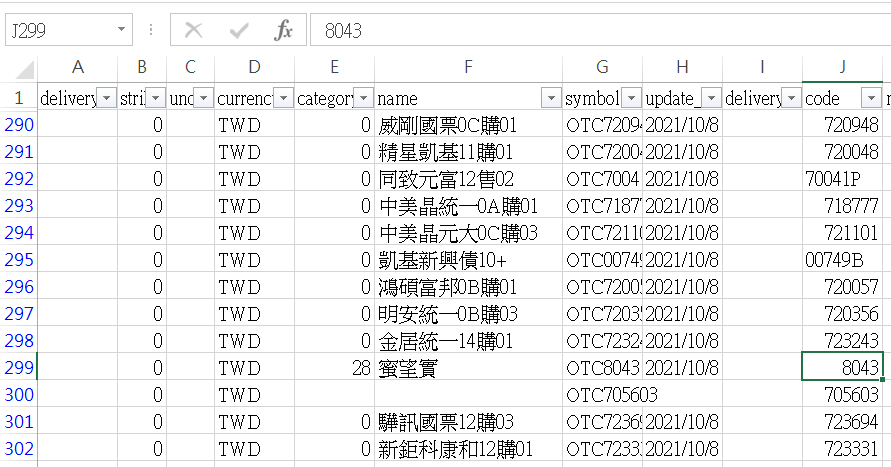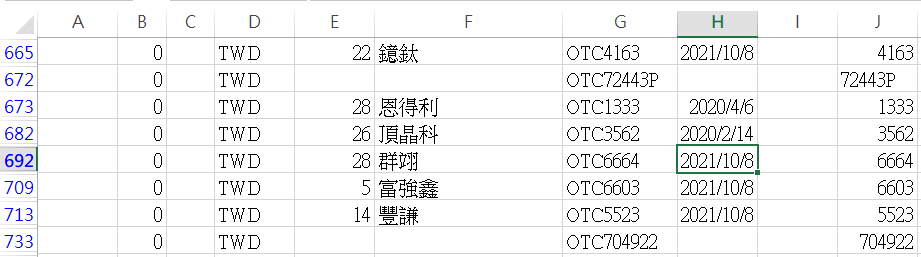``````import os
import shioaji as sj
from shioaji.constant import Exchange
import pandas as pd

api = sj.Shioaji()

person_id=os.getenv('YOUR_PERSON_ID'),
)
print(api.Contracts.Stocks) #確認api.Contracts.Stocks資料已下載完成

stock_list = []

for exchange in api.Contracts.Stocks:
for stock in exchange:
if stock.exchange in (Exchange.TSE, Exchange.OTC):
if stock.category == '00' or stock.category == '':
continue
continue
else:
stock_list.append({**stock})

df = pd.DataFrame(stock_list)
df.to_csv('stock_list.csv', index=False, encoding="utf_8_sig")

api.logout()
``````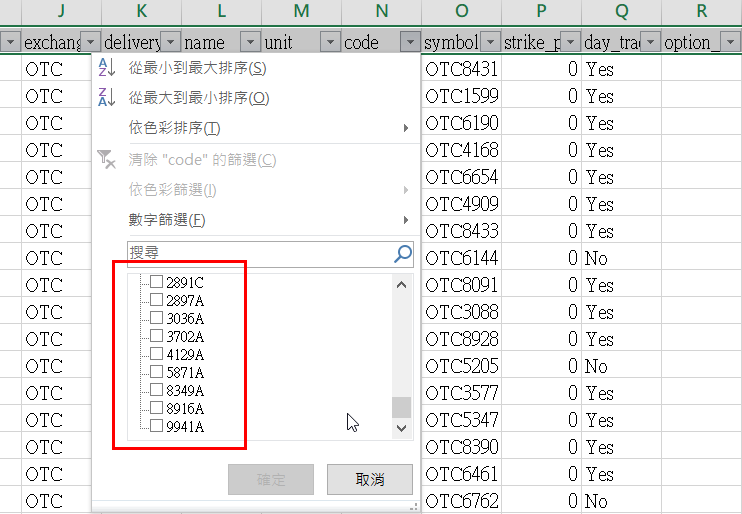``````import os
import shioaji as sj
from shioaji.constant import Exchange
import pandas as pd
import re #匯入re模組

api = sj.Shioaji()
person_id=os.getenv('YOUR_PERSON_ID'),
)
print(api.Contracts.Stocks)
stock_list = []

for exchange in api.Contracts.Stocks:
for stock in exchange:
if stock.exchange in (Exchange.TSE, Exchange.OTC):
if stock.category == '00' or stock.category == '':
continue
continue
elif re.search('[A-Z]', stock.code) is None:
#使用re.search，排除code欄位中有英文字母的Contract
stock_list.append({**stock})

df = pd.DataFrame(stock_list)
df.to_csv('stock_list.csv', index=False, encoding="utf_8_sig")

api.logout()
``````

## 抓取所有股票Kbars資料

``````import os, datetime
import shioaji as sj
from shioaji.constant import Exchange
import pandas as pd
import re #匯入re模組
import sqlite3

TODAY = datetime.date.today().strftime("%Y-%m-%d") #宣告當天日期

conn = sqlite3.connect('D:/shioaji.db') #在D槽底下建立資料庫

api = sj.Shioaji()
person_id=os.getenv('YOUR_PERSON_ID'),
)
print(api.Contracts.Stocks)

for exchange in api.Contracts.Stocks:
for stock in exchange:
if stock.exchange in (Exchange.TSE, Exchange.OTC):
if stock.category == '00' or stock.category == '':
continue
continue
elif re.search('[A-Z]', stock.code) is None:
kbars = api.kbars(stock, start='2018-12-07', end=TODAY)
df = pd.DataFrame({**kbars})
df.ts = pd.to_datetime(df.ts)
df['code'] = stock.code
df.to_sql('stocks_1min_kbars', conn, if_exists='append', index=False)
print(f'{stock.code} {stock.name} kbar data is stored to sqlite')

api.logout()
``````

## 關於1分K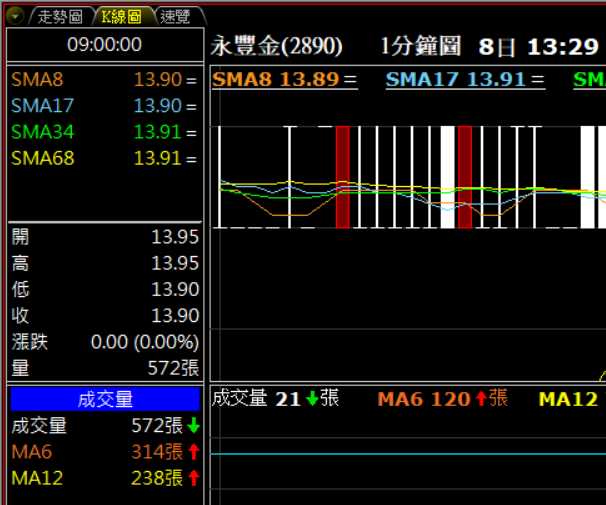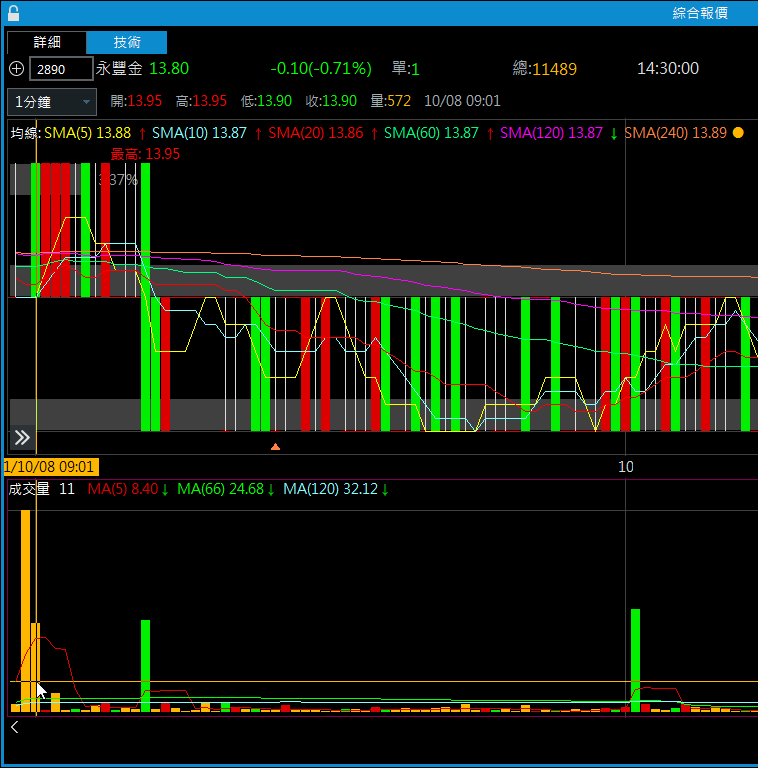``````df.ts = df.ts - pd.Timedelta(minutes=1) #將所有ts的值減1min
``````